Anzeige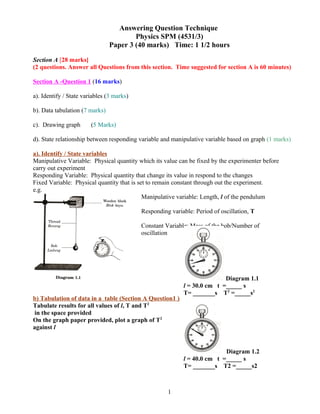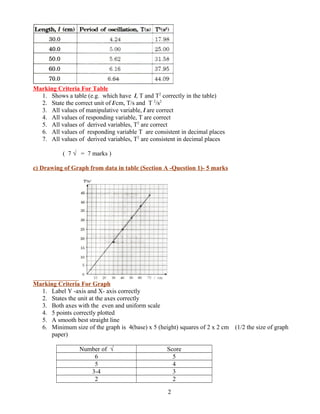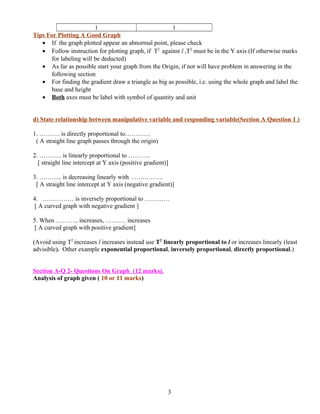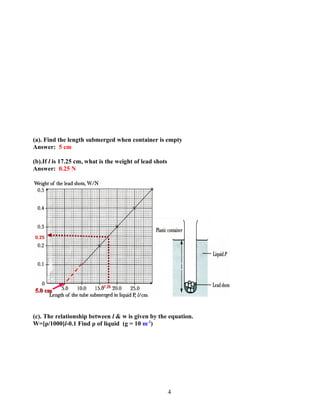Anzeige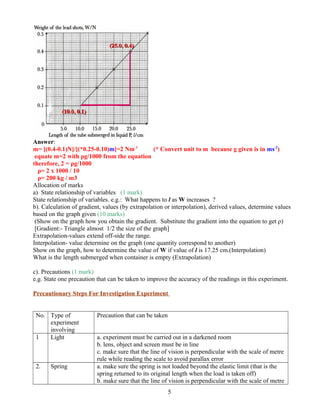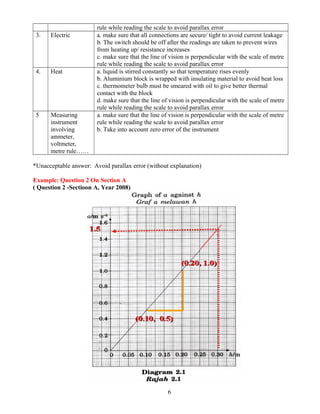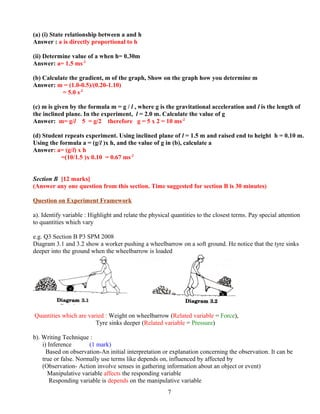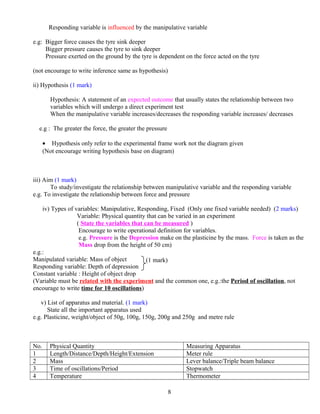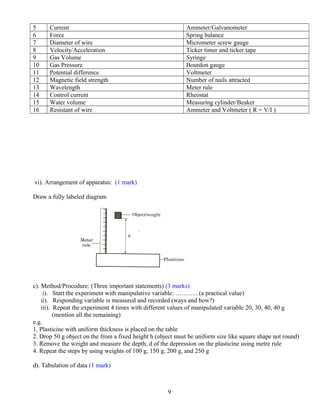Anzeige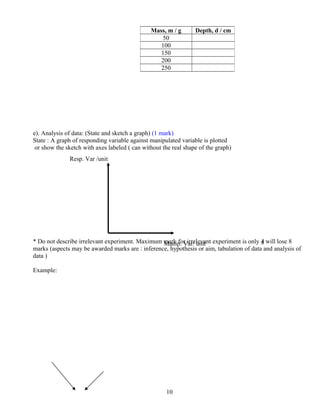Nächste SlideShare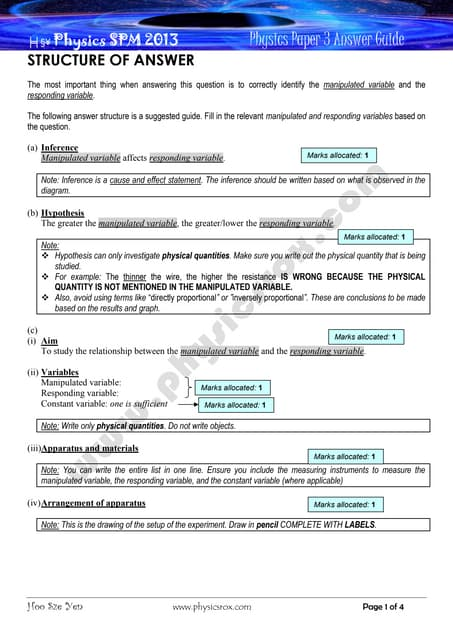SPM PHYSICS-PAPER-3--GUIDE-
1 von 11
Anzeige

### Answering questions technique physics spm paper 3

1. Answering Question Technique Physics SPM (4531/3) Paper 3 (40 marks) Time: 1 1/2 hours Section A [28 marks] (2 questions. Answer all Questions from this section. Time suggested for section A is 60 minutes) Section A -Question 1 (16 marks) a). Identify / State variables (3 marks) b). Data tabulation (7 marks) c). Drawing graph (5 Marks) d). State relationship between responding variable and manipulative variable based on graph (1 marks) a). Identify / State variables Manipulative Variable: Physical quantity which its value can be fixed by the experimenter before carry out experiment Responding Variable: Physical quantity that change its value in respond to the changes Fixed Variable: Physical quantity that is set to remain constant through out the experiment. e.g. b) Tabulation of data in a table (Section A Question1 ) Tabulate results for all values of l, T and T2 in the space provided On the graph paper provided, plot a graph of T2 against l 1 Manipulative variable: Length, l of the pendulum Responding variable: Period of oscillation, T Constant Variable: Mass of the bob/Number of oscillation Diagram 1.1 l = 30.0 cm t =_____ s T= _______s T2 =_____s2 Diagram 1.2 l = 40.0 cm t =_____ s T= _______s T2 =_____s2
2. Marking Criteria For Table 1. Shows a table (e.g. which have l, T and T2 correctly in the table) 2. State the correct unit of l/cm, T/s and T 2 /s2 3. All values of manipulative variable, l are correct 4. All values of responding variable, T are correct 5. All values of derived variables, T2 are correct 6. All values of responding variable T are consistent in decimal places 7. All values of derived variables, T2 are consistent in decimal places ( 7 √ = 7 marks ) c) Drawing of Graph from data in table (Section A -Question 1)- 5 marks Marking Criteria For Graph 1. Label Y -axis and X- axis correctly 2. States the unit at the axes correctly 3. Both axes with the even and uniform scale 4. 5 points correctly plotted 5. A smooth best straight line 6. Minimum size of the graph is 4(base) x 5 (height) squares of 2 x 2 cm (1/2 the size of graph paper) Number of √ Score 6 5 5 4 3-4 3 2 2 2